# Part 3 : Following along MIT intro to deep learningAbhijit Ramesh / March 16, 2021

13 min read––– views

## Introduction

Vision is one of the things that most of us humans take for granted, there are many information that out brain processes due to vision. Things like complex human emotion, predict incoming danger. Vision help us drive vehicles and much more.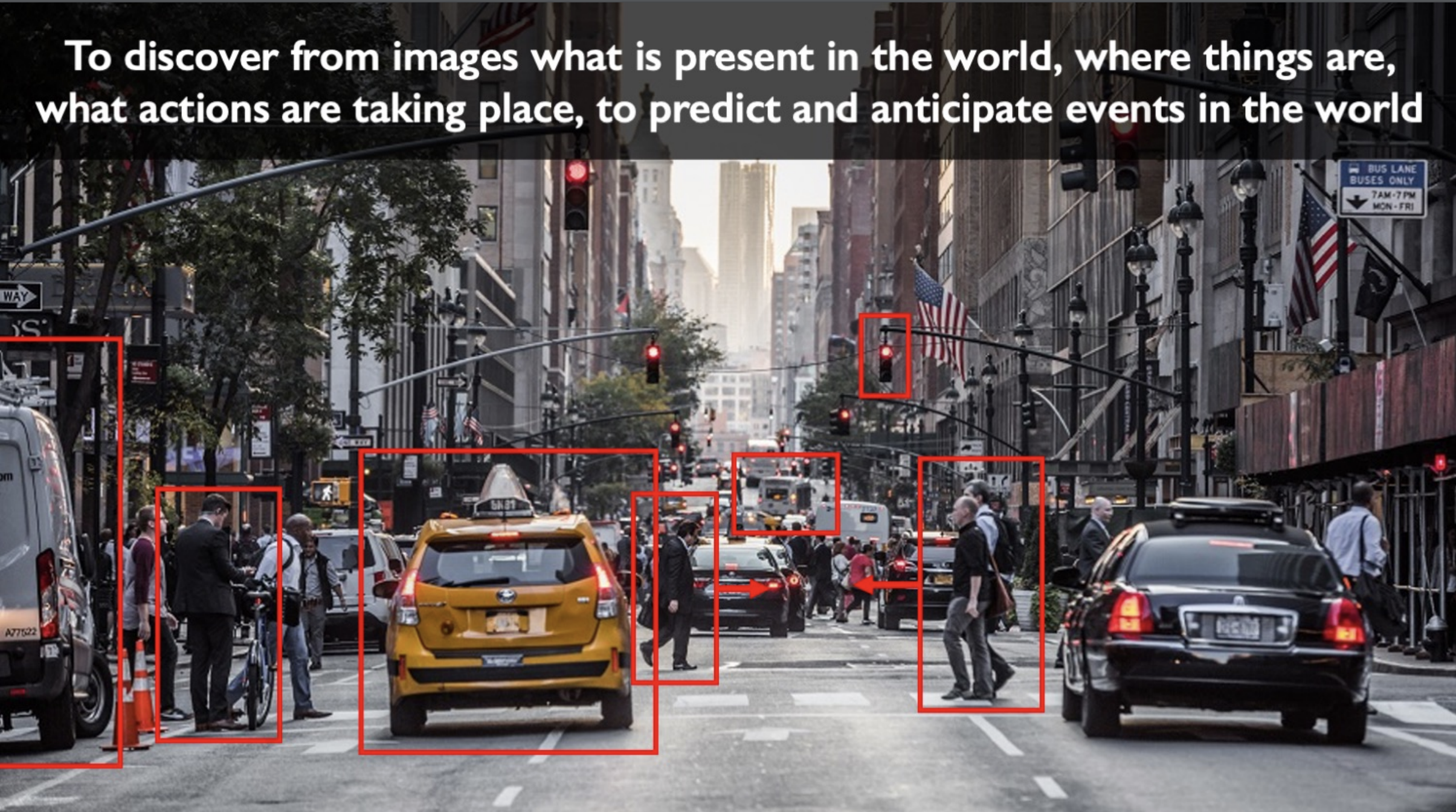Take a look at this image we can make lot of inference from this image for example our brains automatically pays more attention to the yellow car more than the white truck on the left because we know that the white truck is not going to move anytime soon because it is parked and the taxi might also not likely to be moving because there are pedestrians walking by. Our brain also processed information in the background such as the traffic light which is upfront and knows that the traffic is most likely not going to move. It can also make some sense of the images way up in the road as well. This is why I said most of up take this sense for granted not because we don't appreciate it but because our brain does so much processing in the split of a second and make decision on how we drive.

In real word making a computer do these tasks are a very tedious task and especially if you are planning to follow standard programming methodologies without using any form of AI. But due to the advancements in the field of deep learning these techniques have become relatively easy to tackle.

## The rise and impact of computer vision

Computer vision is everywhere right now,

Everything form making robots that can take in some visual cue and perform some task.

The facial recognition on our phones.

Vision is also used in biology and medicine to detect diseases such as cancer.

Computer Vision algorithms are also advantages to visually impaired people as it has improved accessibility in some applications.

One of the most celebrated applications of computer vision is none other than autonomous driving.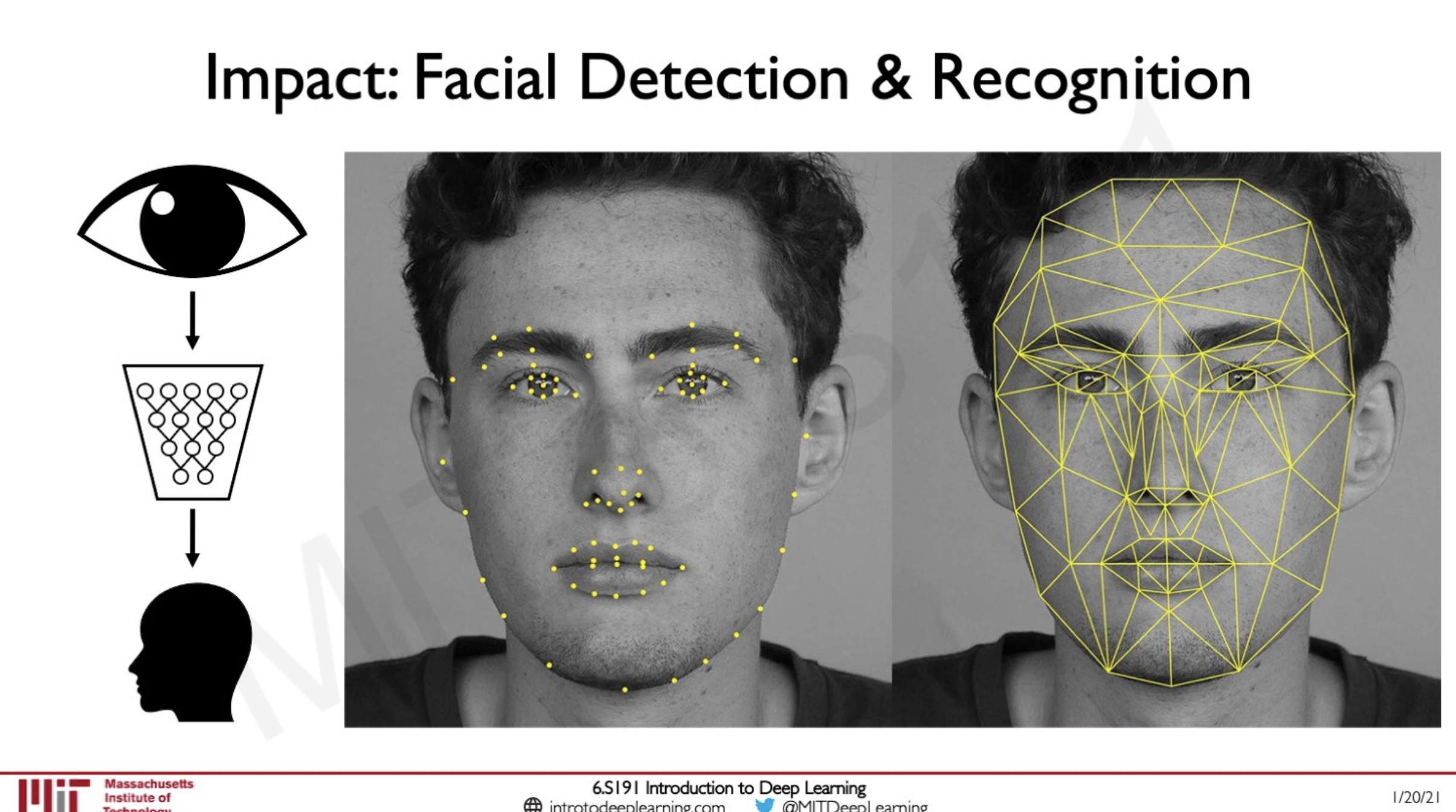Here we are mimicking sort of something our brain would be doing looking at a person to identify who it is this seems like an easy task for us humans but if we put a neural network to this task this might be something that we are not expecting to be easily done it might be looking at things like different features, contours and even an outline of the facial features.

We can also use the same technique to some other task instead of identifying a person lets say it has to determine driving control for steering given what the human eye sees on the road.

Vision is also used in taking MRI scans from and passing it through a neural network to check for some disease like Breast cancer or given the images of skin sample to see if there is skin cancer and now-days given images of the lungs to determine if a person is suffering from COVID-19.

The above video shows a story of how computer vision helped visually impaired person to run.

## What computers "See"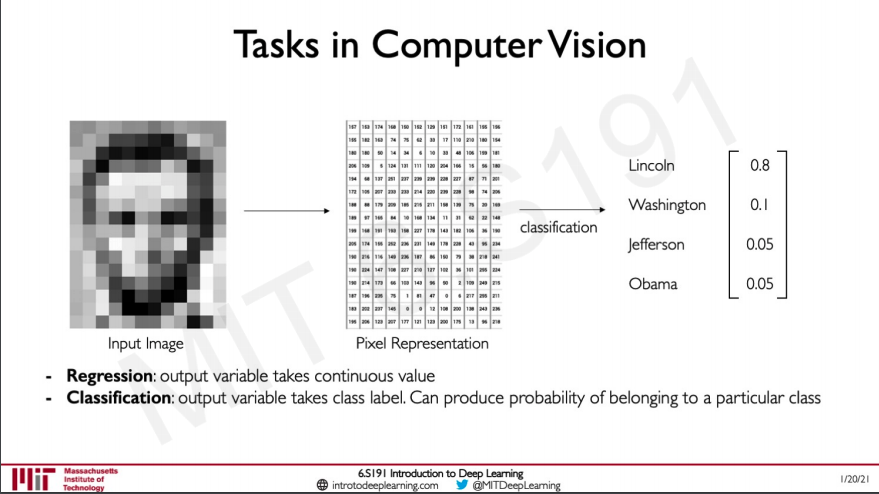Computers represent images as numbers, Images are made up of pixels and these pixels are represented by numbers in the case of a grey scale image these pixels will have one value. In the case of a colour image, this would be 3 values reach representing Red, Green and Blue channels.

There are generally two tasks in computer vision either to classify the image that is predict if they belong to a particular pre-defined class this is classification. Then there is Regression that is output a continuous value.

How do these networks classify and image ?

It looks for features in the image category like for example in a face it would be noes, eyes, mouth. In a car, it would be wheels, license plate and headlights. In the image of an house it would be a door, windows and steps.

### Manual Feature Extraction

While manual feature extraction is possible it is a hard problem to tackle since there are lot of problems that could come into play as shown above. The network should be able to handle these problems and not be effected by such effects such as changes in lighting condition or background clutter etc...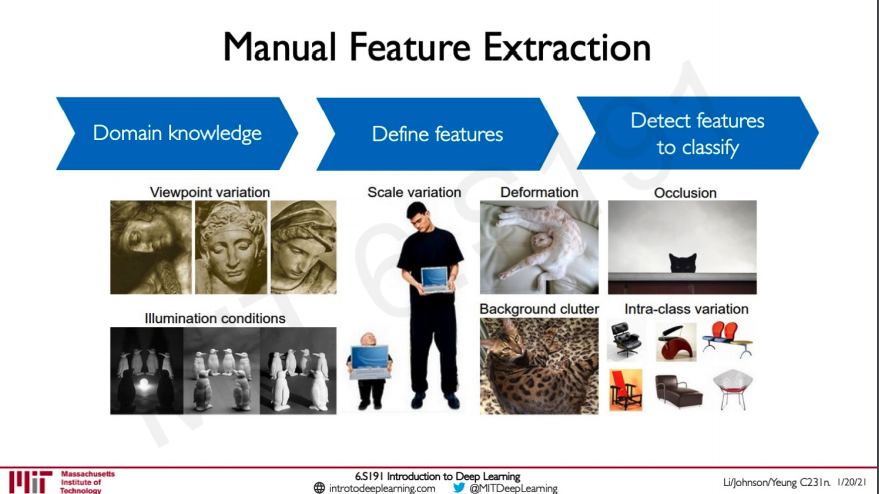The way of solving it is by learning feature representations that is first learning some low level features and then building on top of these from hierarchical data this would allow the network to learn feature without being explicitly told to do so.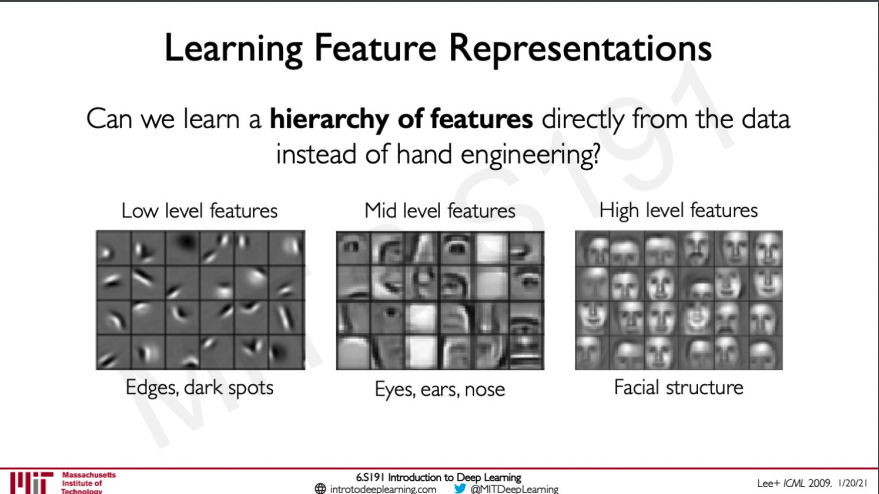## Learning Visual Features

In order to appreciate how good CNNs are we need to first understand what happens when we pass an image to a fully connected network.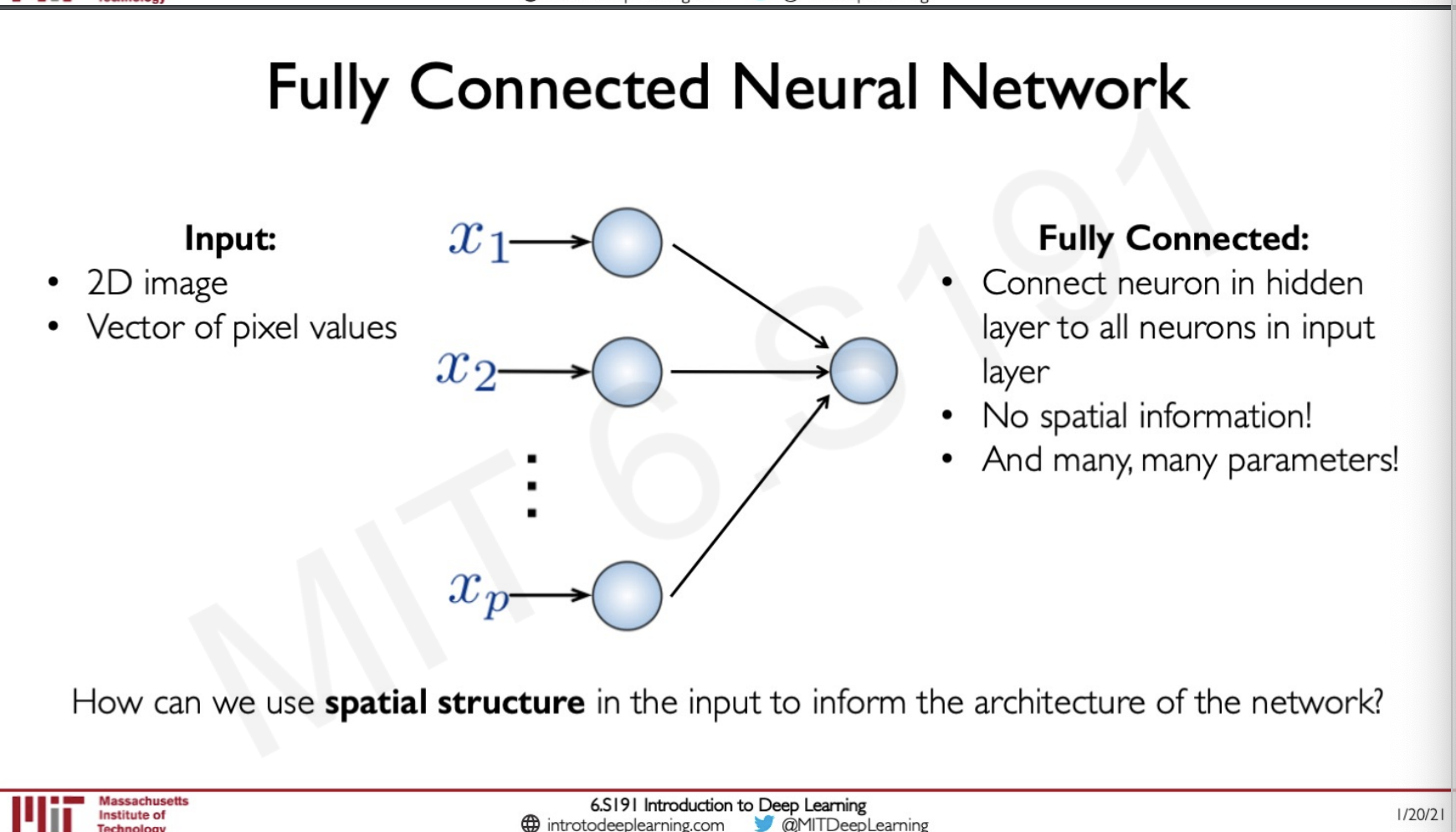In order to pass a 2D image to a fully connected network we need to flatten the image first and what this means is that stacking the dimensions on top of each other and then passing it to the FCN. The problem is that when we do this any spatial information is lost and as we know in an image a pixel and its neighbours would have a lot of information which could be learned by the neural network but because of the problem that the spatial information is lost this would be a hard task. Since we have lot of learning to do here now the parameters will also keep on increasing.

So how do we keep the spatial structure ?

We create connected patches in input layer to a single neuron in the subsequent layer. We slide these patches through the whole image to define connections. These patches have weights associated with them these weights are used to extract local features.

A particular set of weights (a filter) extract a particular set of features.

We use multiple filters to extract different features.

These filters share parameters spatially so features that it is easy to identify features that matter in one part of the input that should matter elsewhere.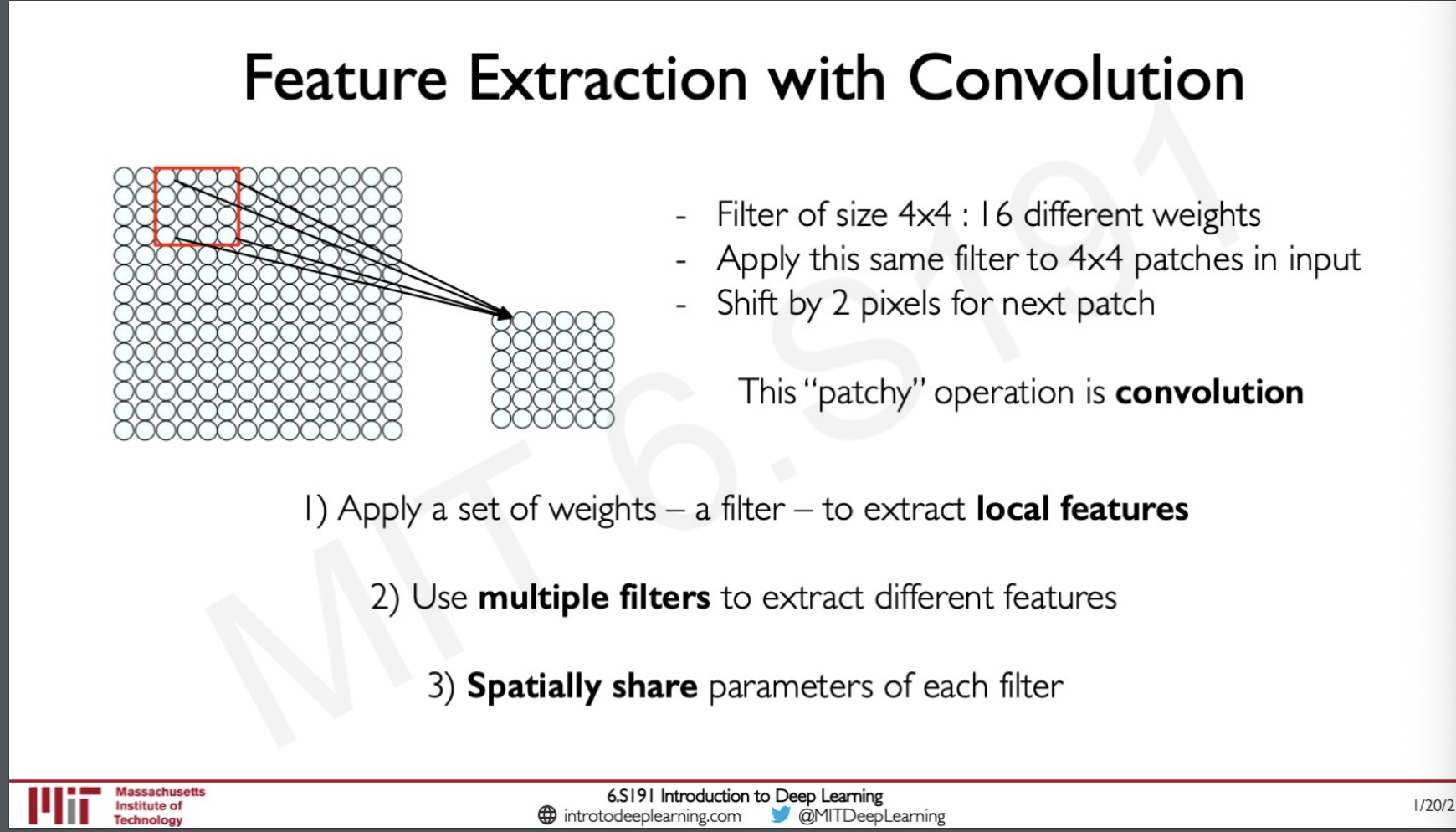## Feature Extraction and Convolution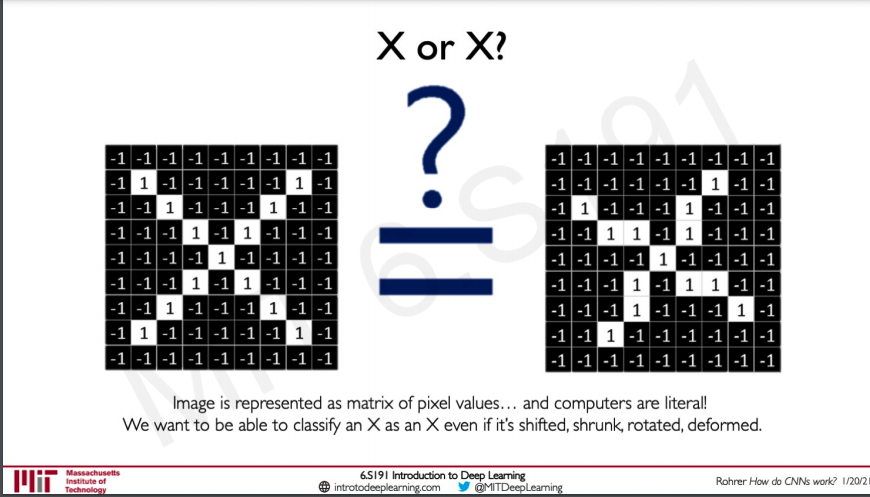As we have talked about earlier manually engineering features will not work out in image classification for example see the two images above they are both X regardless of if one is tilted by a bit or shifted a bit to the side and whatnot so that means we cannot simply put the matrices on top of each other and determine if they are X or not instead we should look for the features in the patches.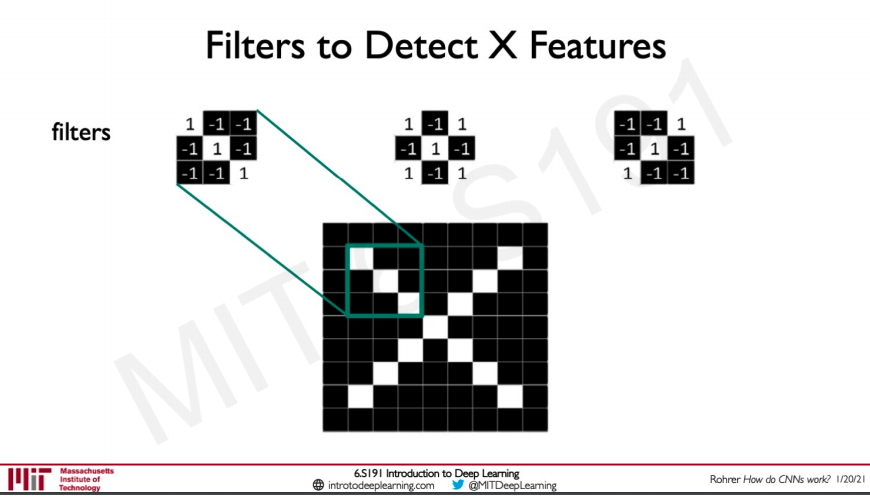As we can see here the filters actually represent some features like a diagonal on the left arm of the image or the intersecting diagonals in the middle of the image this would allow for easier comparison of the features across the images.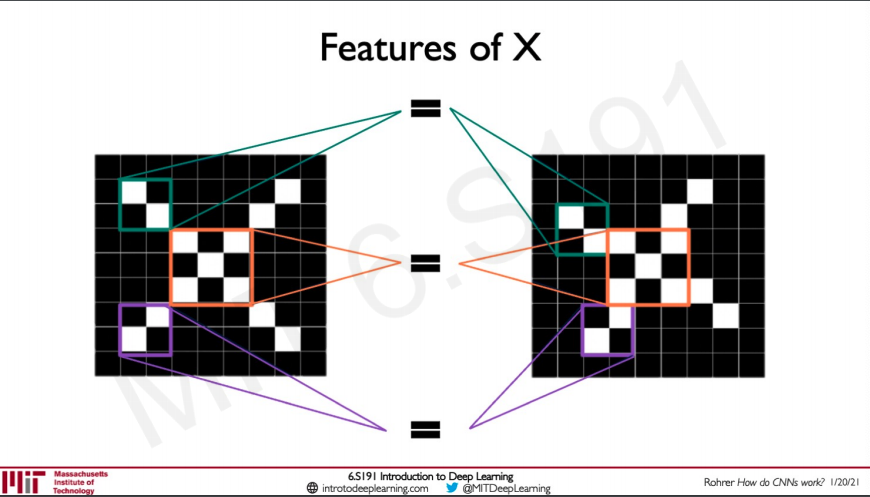So how does the network figure out where this feature is occurring in the image, this is done with the help of a convolutional operation.

## Convolution Operation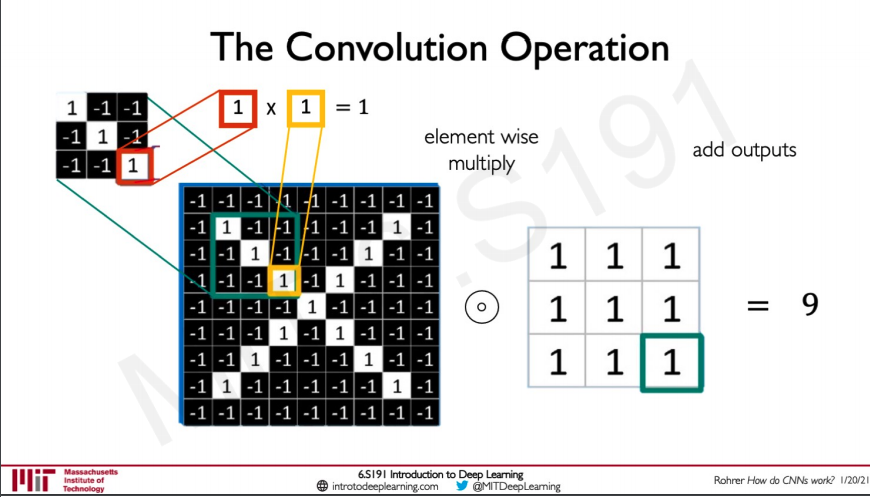Convolution operation is basically taking a patch of image and then doing element wise addition between them this would result in a new matrix and we add all the elements in this matrix together to get an output.

So how does this happen over an image ?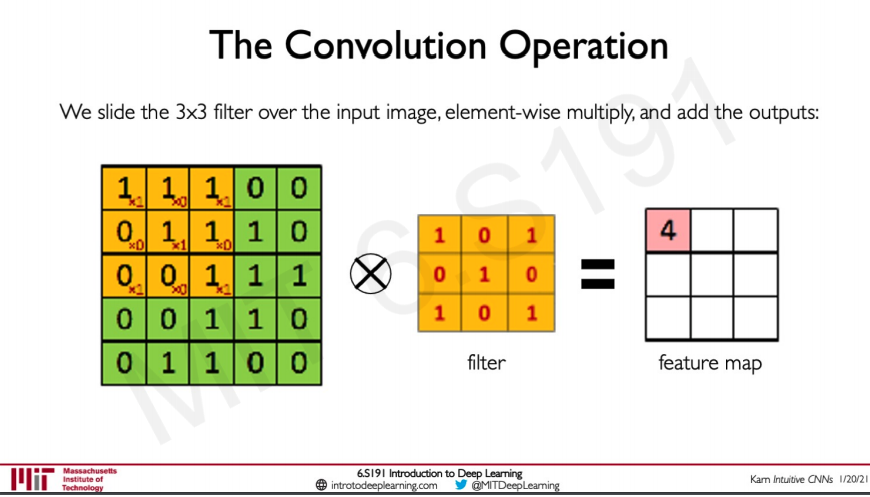We slide the filter over these images doing the said operation over and over again until we have a feature map.

The next slide would look like this,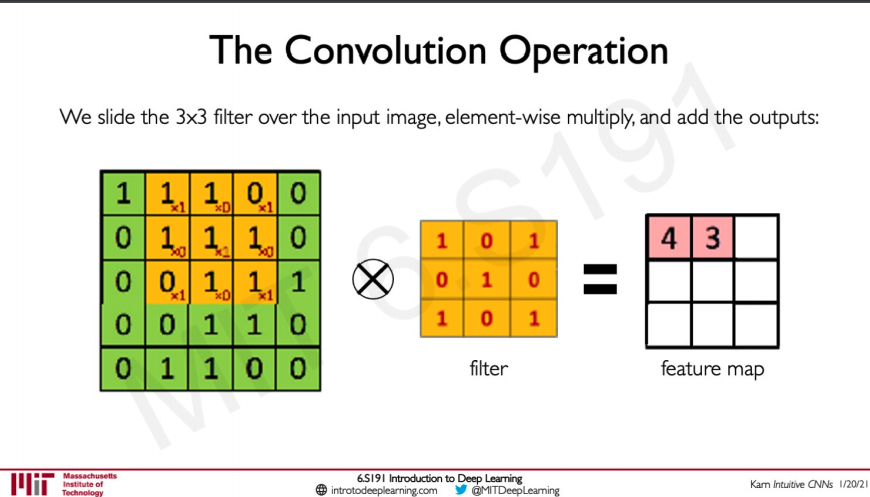These filters have nothing but weights we can set these weights to a particular value to detect a particular feature.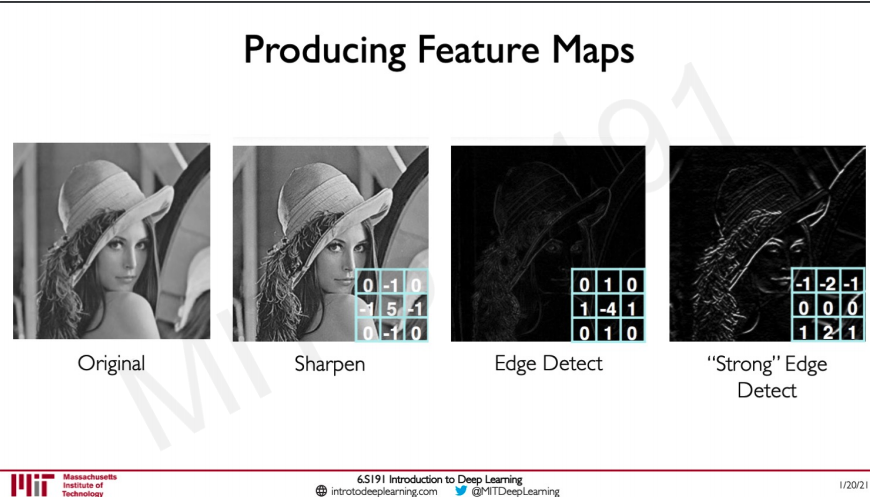Here we can see how different values of the weights can help in identifying different features in an image.

The thing about Neural Networks is we don't have to hand engineer these features they automatically learn what pattern to look for in these images and change the weights accordingly.

## Convolutional Neural Networks (CNNs)

The CNN architecture consist of three main layers

1. Convolution - This applies a filter to generate feature maps
2. Non-linearity - To deal with non-linear data; Often ReLU
3. Pooling - Downsampling operation on the feature map to feed to a fully connected layer.

### Convoltional Layers

Let us see mathematically how the convolution step works,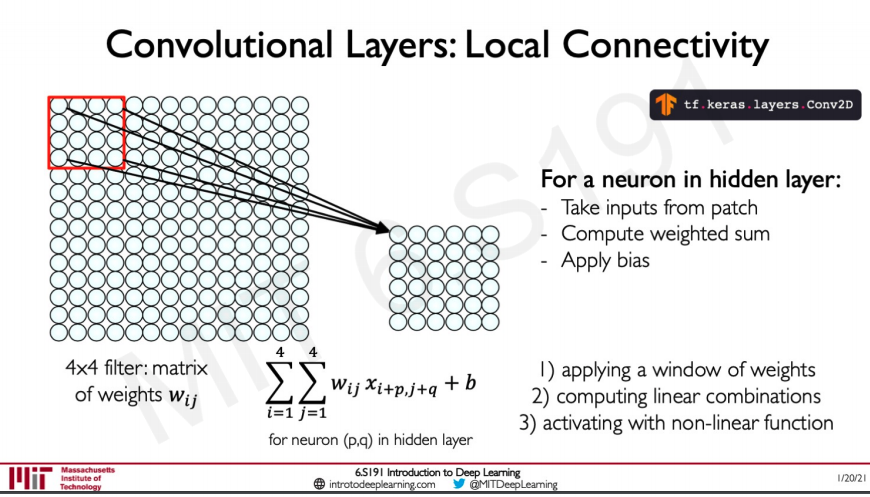The networks,

• Takes a patch from the input image
• Perform element wise multiplication to the weights
• Compute the weighted sum on the ouput
• Finally applies an activation

Mathematically this is how it would look like on a kernel of size 4x4

$\sum_\bgroup i=1\egroup^\bgroup 4 \egroup \sum_\bgroup j=1 \egroup^\bgroup 4 \egroup W_\bgroup ij \egroup x_\bgroup i+p,j+q\egroup + b$Here p and q are the hidden layers The sum is done across the row and column for the matrix of size 4 from i=1 to 4 and j=1to4 where the weights are represented by $W_\bgroup ij \egroup$ and the input is represented by $x$.

b is the bias so that we can shift the non-linearity left or right.

When we construct this feature map from the images we need to now think about the volume of the output we are creating a layer with dimensions h x w x d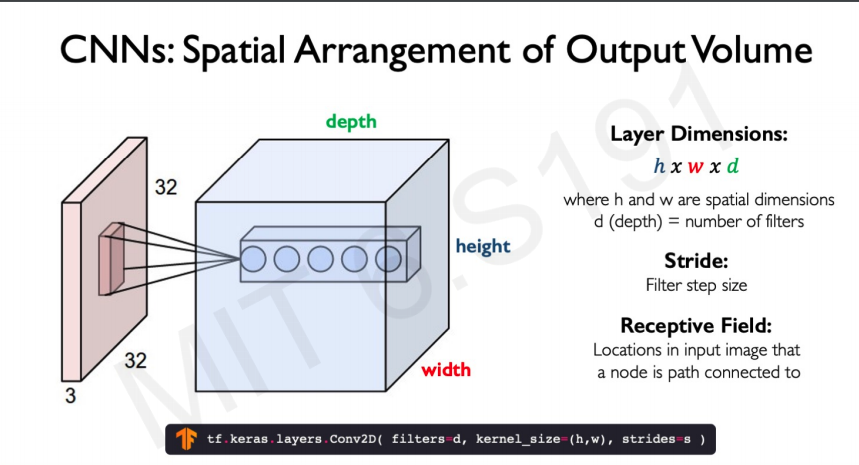### Non Linearity

We need to apply non linearity after each layer in the CNN this is because image data is non linear and we need to keep on apply these non linearities so that the images are smooth.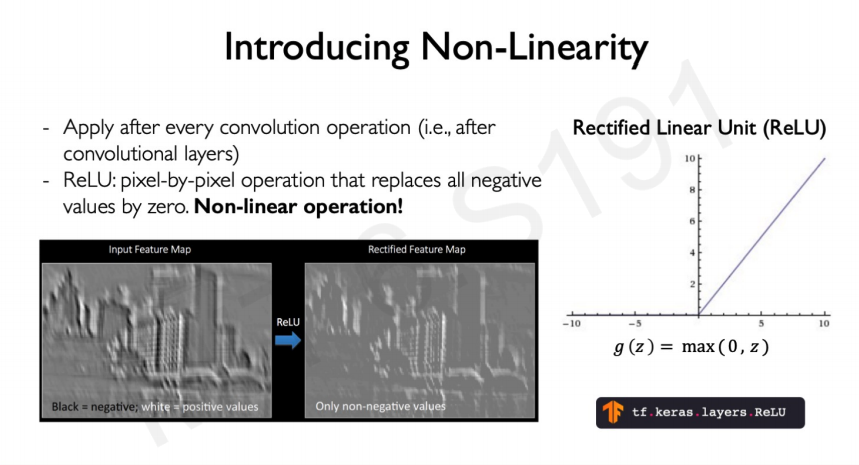A very commonly used non linearity is ReLU this takes all the negative values and change it to zero while keeping the positive values as it is. We can see how ReLU helps in the above image.

### Pooling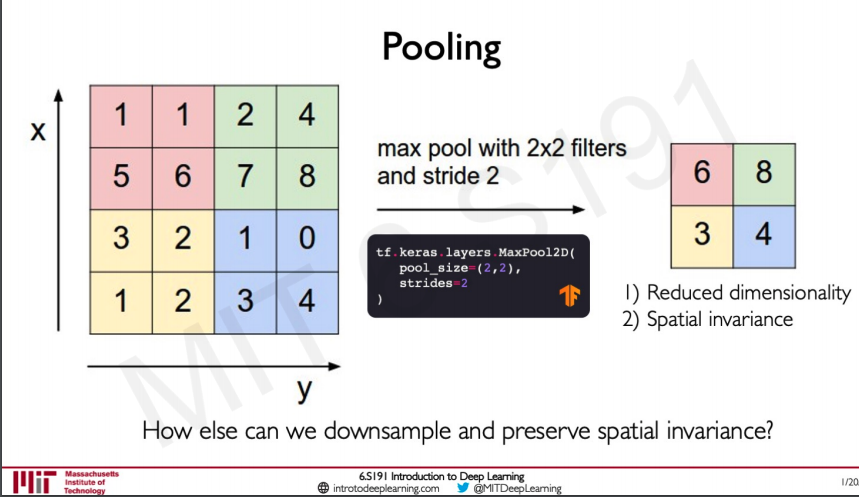There are many kinds of pooling operations the main use of pooling is to reduce the dimensionality of the layer while keeping some of the spatial invariances, most commonly used pooling is max-pooling which takes the maximum value of a specified pool size example a pool size of 2x2 will take the max value from the matrix of 2x2 which would be striding with 2 steps as we can see above.

Now we know everything that happens in a convolution layer once we have done this repeatedly we should pass this on to a fully connected layer after flattening the output of the last convolution layer in our network.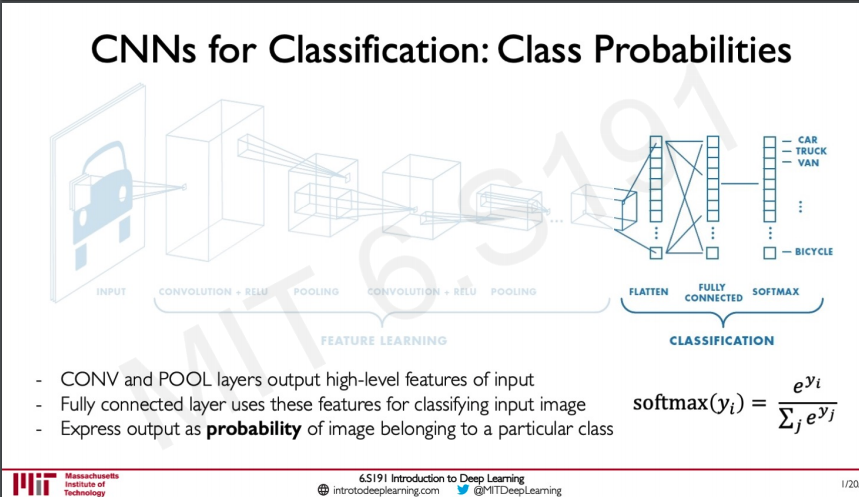Generally, if we are doing classification on image data we use a softmax activation function so that we get the output as a probability of the given input is a particular class.

## Putting it all together

import tensorflow as tf
def generate_model():
model = tf.keras.Sequential([
# first convolutional layer
tf.keras.layers.Conv2d(32,filter_size=3,activation='relu'),
tf.keras.layers.MaxPool2D(pool_size=2,strides=2),
# second convolutional layer
tf.keras.layers.Conv2d(64,filter_size=3,activation='relu'),
tf.keras.layers.MaxPool2D(pool_size=2,strides=2),

# fully connected classifier
tf.keras.layers.Flatten(),
tf.keras.layers.Dense(1024,activation='relu'),
tf.keras.layers.Dense(10,activation='softmax')
])
return model

        # first convolutional layer
tf.keras.layers.Conv2d(32,filter_size=3,activation='relu'),
tf.keras.layers.MaxPool2D(pool_size=2,strides=2),


In the first convolution layer, we are defining a feature map of size 32 and apply an activation relu. We are also doing MaxPooling here to downsample the dimensions. Then we feed this feature map to the next set of convolution and max-pooling layer.

        # second convolutional layer
tf.keras.layers.Conv2d(64,filter_size=3,activation='relu'),
tf.keras.layers.MaxPool2D(pool_size=2,strides=2),


After this we flatten the output and pass it on to a dense layer with 1024 units.

        # fully connected classifier
tf.keras.layers.Flatten(),
tf.keras.layers.Dense(1024,activation='relu'),
tf.keras.layers.Dense(10,activation='softmax')


Since we are classifying to 10 classes we would be changing the final year to have 10 units and as said before doing a softmax activation to it as well.

## An Archtecture for Many Applications

Since these CNNs are very good feature extractors we can use this on wide variety of applications if we think of it as something like a having two side one side for feature extraction and another to perform some task.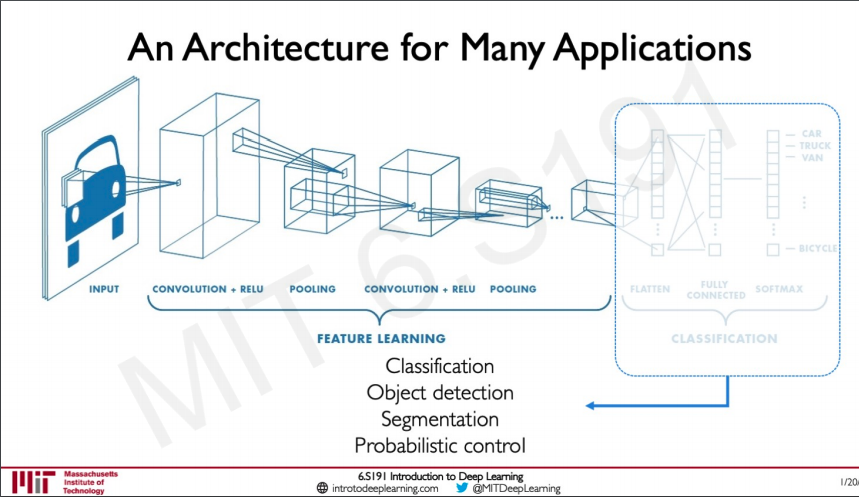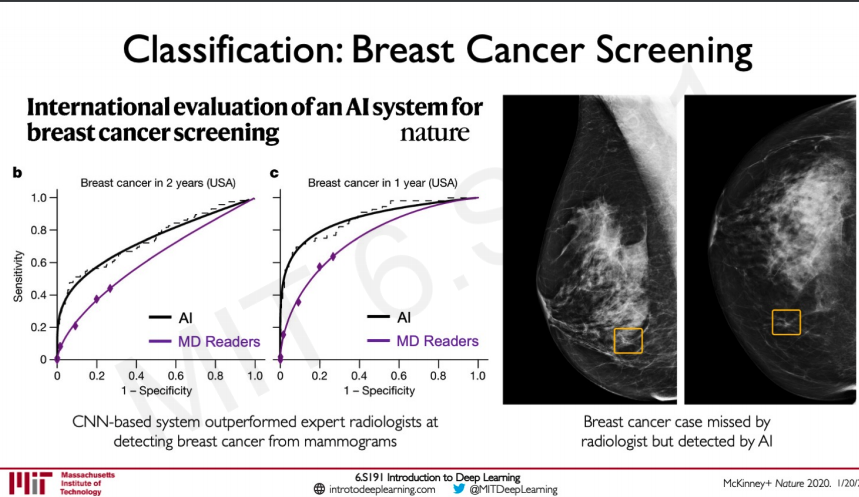Here the performance from a paper on how CNN has been used to train on complete medical image data is shown.

## Object Dectection

A more challenge task we can do with CNN is object detection.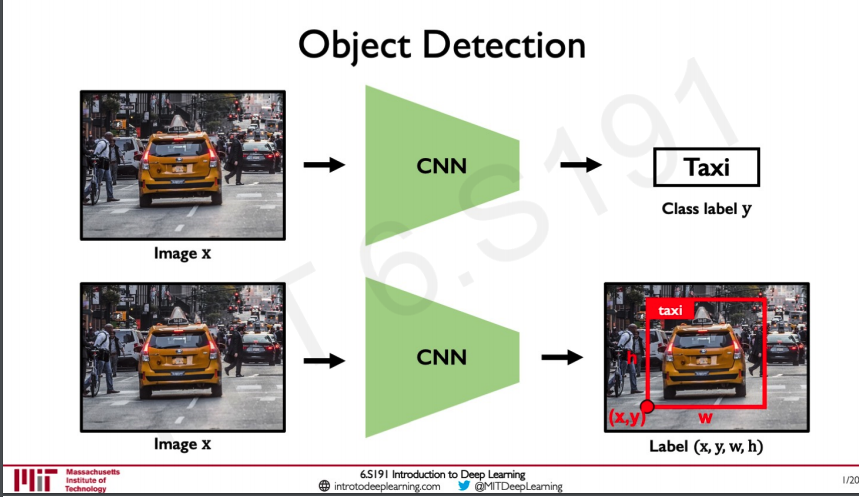What we have seen now is to predict if the image belongs to a particular class but what if we should put a bounding box around the image and also predict what the image is.

Here we need to know where the image is

What height and width the image has and also predict what the bounding box contain.

A tougher challenge would be to do this given more object to deactect.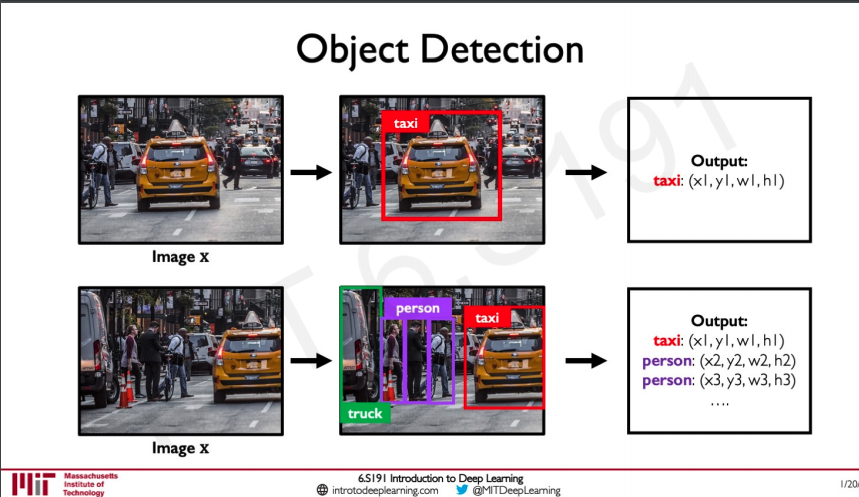Here we can see there are other classes like truck person etc...

### Naive Solution

The naive way of solving this would be to randomly draw a box in the image and see if there is the CNN is able to predict a class there if so that means we can leave the bounding box there and we detected an object.

But there are a lot of problems with this there are way too many inputs too many scales, positions and sizes.

### Object Dectection with R-CNNs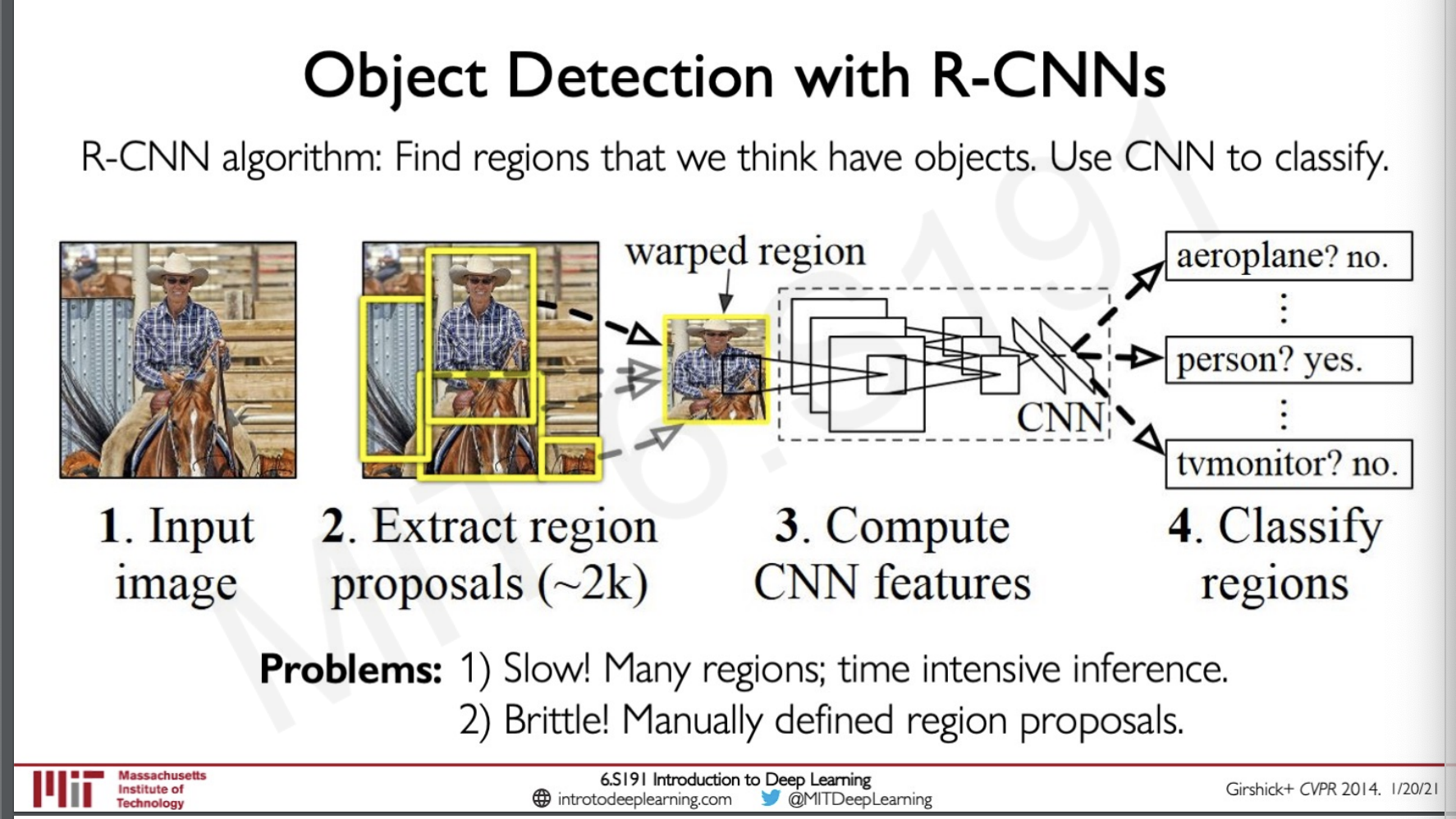Here we use some form of heuristic and manually define regions which we might think the CNN might be able to find some features on and the rest is the same we pass it to a classifier to determine if we have some class or not.

This is also a slower approach and involve lot of manual work.

And still we are passing the image multiple time through the network to do classification.

## Faster R-CNN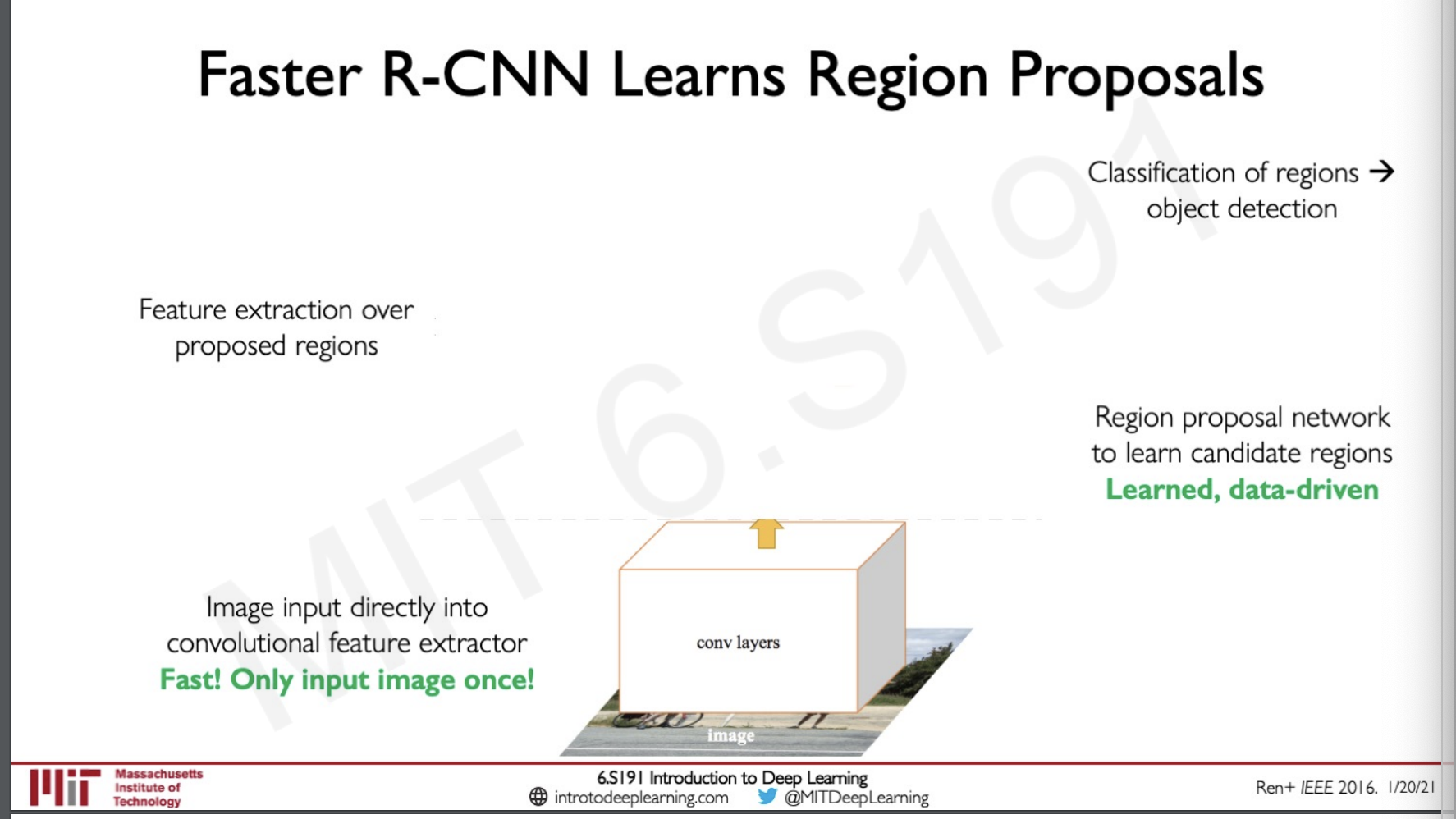In this approach we only pass the image once but here we first have a network which does the job of predicting what regions the classifier might be interested in or where to draw the bounding boxes on and this part is also trained from data. Then we can attach it to a classifier to do the prediction of what class the particular object belong to.

## Semantic Segmentation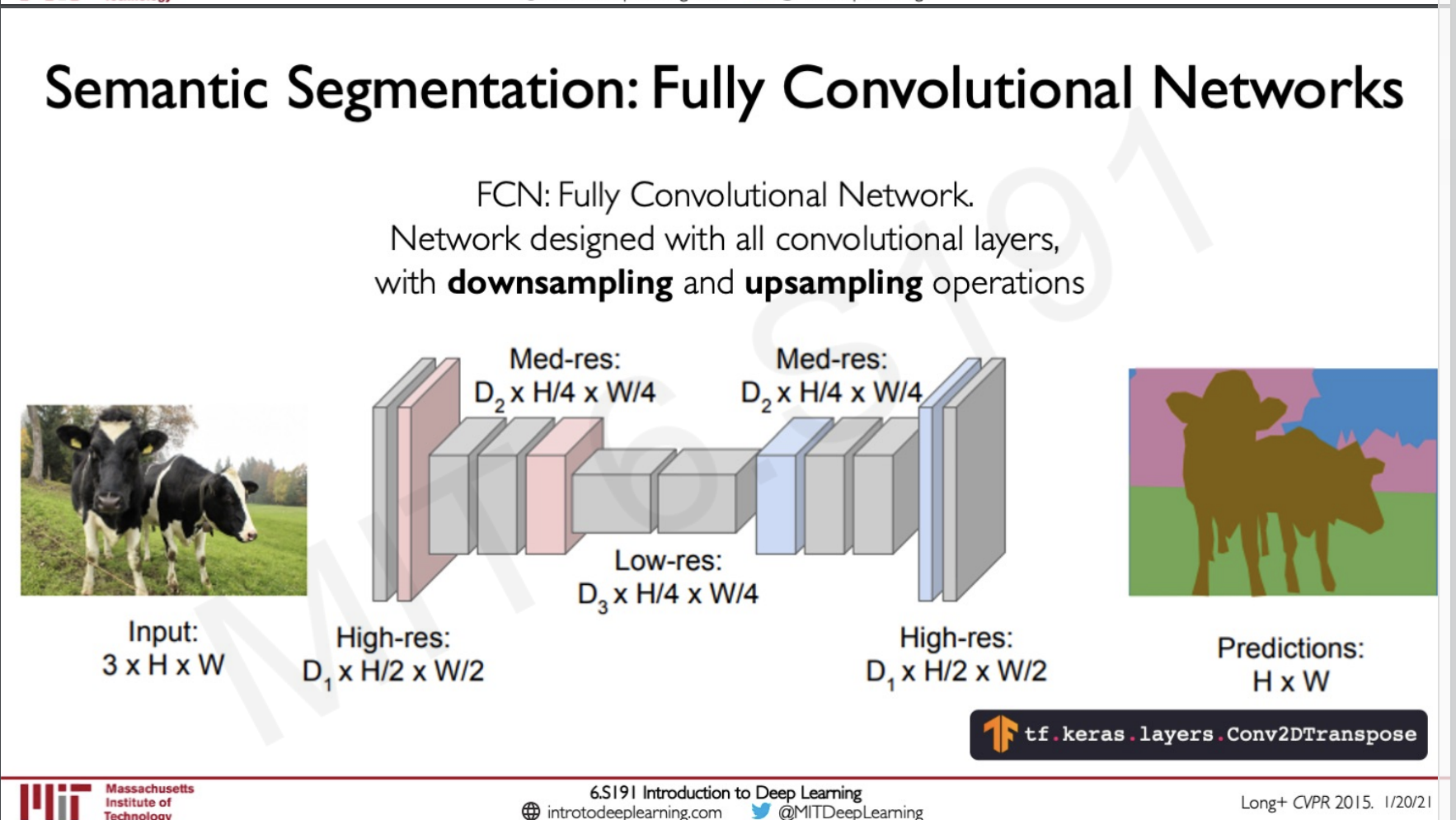This is a more advanced application of CNN where an image is passed through the network and the output is also an image but with segmentation where a segment is attributed to a colour representing a class.

Semantic segmentation is also used in biomedical image analysis.

## Continuous Control: Navigation from Vision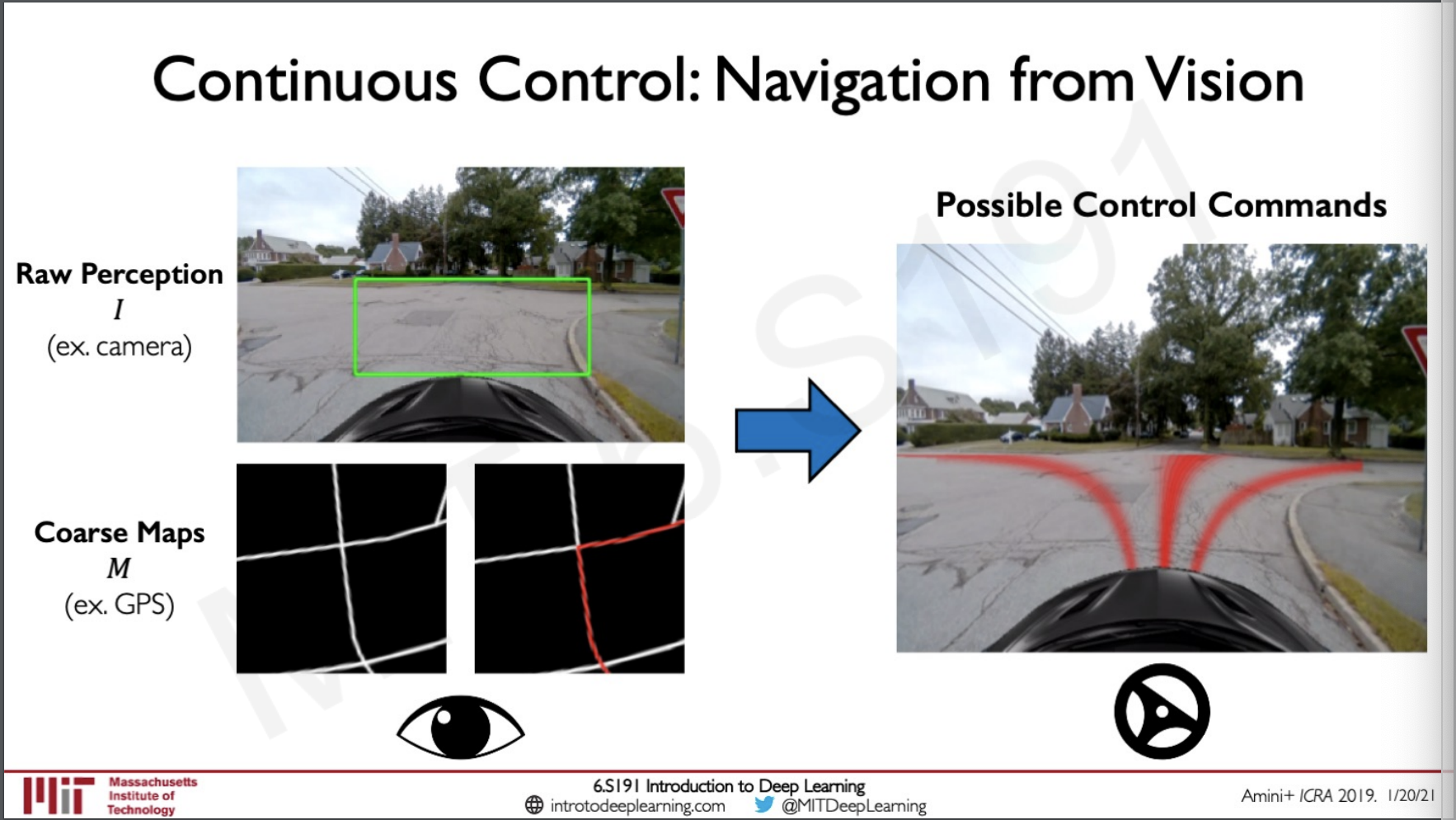Instead of tasking CNN with let's say doing just classification we can also make to do some task like predicting possible control commands for driving a car given the raw image from the cameras as well as a course map overview from google images. Here the model would be predicting over a whole probability distribution.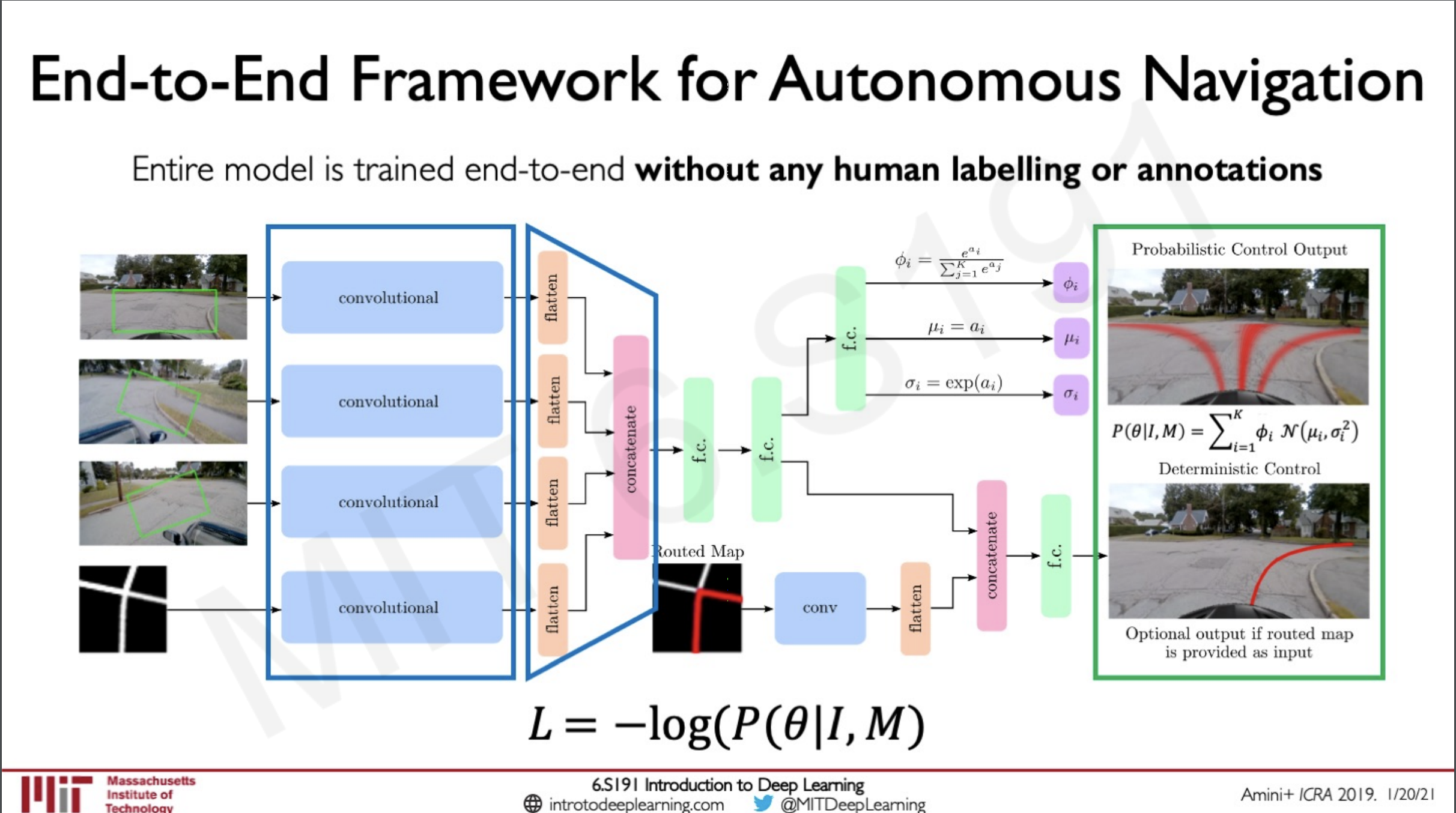How these models achieve this is by having models that are trained end to end with concatenation layers in addition to the layers we have seen like convolution, fully connected max-pooling etc.. and being able to have a single deep neural network that can be used end to end to perform the said task.

## Source

MIT intro deep learning : http://introtodeeplearning.com/

Slides on intro to deep learning by MIT :

http://introtodeeplearning.com/slides/6S191_MIT_DeepLearning_L3.pdf

## Lab Solutions

https://github.com/abhijitramesh/learning-introtodeeplearning/blob/main/lab2/Part1_MNIST.ipynb

##### Subscribe to the newsletter

Get emails from me about machine learning, tech, statups and more.

- subscribers